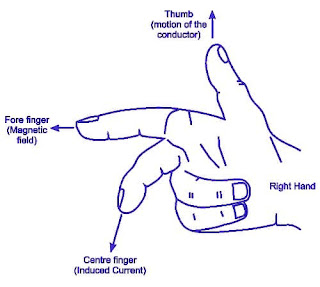# Fleming's left-hand rule and Right hand rule-Motor and Generator

When the current-carrying conductor placed in a magnetic field, it experiences a force due to the magnetic field on the other hand if the conductor moved into the magnetic field an emf gets induced across the conductor as per faraday's law. Hence the direction of the force and the direction of induced emf current can be determined using fleming's left-hand rule for motor and Fleming's right-hand rule for generator.

## Fleming's left-hand rule

The direction of the force experienced by a current-carrying conductor kept in the magnetic field can be determined by the rule called fleming's left-hand rule.Fleming's left-hand rule

Fleming's left-hand rule can be stated as stretch out the three fingers of the left hand, namely the forefinger, middle finger, and thumb. such that they are mutually perpendicular to each other. Now point the forefinger in the direction of the magnetic field and the middle finger in the direction of the current then the thumb indicates the direction of the force experienced by the current-carrying conductor. Fleming's left-hand rule is used to determine the direction of a force in the motor.

The magnitude of force experienced by the conductor depends on the following factors:
• Flux density(Wb/m2) of the magnetic field in which the current-carrying conductor placed.
• The magnitude of the current passing through the conductor
• Length of conductor
F=BIl Newton

## Fleming's Right-hand rule

The direction of induced emf can be decided by using Fleming's right-hand rule as the left-hand rule is used to determine the direction of force experienced by the current-carrying conductor placed in the magnetic field, Fleming's right-hand rule is used to get the direction of induced emf when the conductor is moving in a magnetic field.Fleming's Right-hand rule

Fleming's right-hand rule defines as stretch out three fingers of the right hand namely the thumb, forefinger, and middle finger as perpendicular to each other. point the forefinger of the right hand in the direction of flux lines and the thumb in the direction of motion of conductor with respect to flux then the middle finger will point in the direction of induced emf.